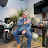# How LENZ’S LAW Invalidates The LAW OF CONSERVATION OF ENERGY & Proves The LAW OF CREATION OF ENERGYLenz’s Law if Induction establishes that all electric generators perform Negative Work with Electromagnetic Field Energy that is created. https://www.slideshare.net/PDiCEOThan…

WORK – ENERGY – POWER & THE WORK-ENERGY PRINCIPLE http://hyperphysics.phy-astr.gsu.edu/…

WORK: refers to an activity involving a force and movement in the direction of the force.

ENERGY: is the capacity for doing work. You must have energy to accomplish work – it is like the “currency” for performing work.

POWER: is the rate of doing work or the rate of using energy.

WORK-ENERGY PRINCIPLE: The change in the kinetic energy of an object is equal to the net work done on the object. In 1834 Lenz’s Law, named after the physicist Emil Lenz, confirmed the Law of Creation of Energy in electricity generation. Lenz’s Law states that the direction of the electric current which is induced in a conductor by a changing magnetic field according to Faraday’s Law of Induction is such that the Magnetic Field Energy created by the induced current will perform Negative Work and oppose (reduce the Kinetic Energy) of the initial changing magnetic field.

Law of Creation of Energy History 1820-2021 https://www.slideshare.net/PDiCEOThan…

1. Law of Creation of Energy History 1820 – 2007 In 1820 Hans Christian Ørsted discovered the Law of Creation of Energy when an electric current was passed through a wire, the Magnetic Field Energy created around the wire would perform Work on a compass needle and cause the Kinetic Energy of the needle to increase.

2. In 1822 Michael Faraday expanded application and confirmation of the Law of Creation of Energy when he employed created Magnetic Field Energy in his Electric Motor apparatus – where the Magnetic Field Energy performed Work on a wire (increasing its Kinetic Energy) and caused it to rotate around a magnet submerged in a vessel of Mercury.

3. In 1827 German physicist Georg Ohm, formulated Ohm’s Law which described measurements of applied voltage and current through simple electrical circuits containing various lengths of wire. Ohm’s law states that the current through a conductor between two points is directly proportional to the voltage across the two points.

4. The Power Rule can be used to determine how much Electrical Energy is being converted to Heat Energy in a current bearing wire. For example; in the circuit below the Electric Energy being converted to Heat Energy or the Work performed in that conversion is 48 Watts.
Voltage [ V = I x R ] = 2 x 12Ω = 24V
Current [ I = V ÷ R ] = 24 ÷ 12Ω = 2A
Resistance [ R = V ÷ I ] = 24 ÷ 2 = 12 Ω
Power [ P = V x I ] = 24 x 2 = 48W

5. The Power Rule can also be used to confirm the Law of Creation of Energy and to determine how much Electrical Energy is being converted to Magnetic Field Energy in a current bearing wire.

For example; in the circuit below the Electric Energy being converted to Magnetic Field Energy or the Work performed in that conversion is 0 Watts.
Voltage [ V = I x R ] = 2 x 12Ω = 24V
Current [ I = V ÷ R ] = 24 ÷ 12Ω = 2A
Resistance [ R = V ÷ I ] = 24 ÷ 2 = 12 Ω
Power [ P = V x I ] = 24 x 2 = 48W

6. In 1834 Lenz’s Law, named after the physicist Emil Lenz, confirmed the Law of Creation of Energy in electricity generation. Lenz’s Law states that the direction of the electric current which is induced in a conductor by a changing magnetic field according to Faraday’s Law of Induction is such that the Magnetic Field Energy created by the induced current will perform Negative Work and oppose (reduce the Kinetic Energy) of the initial changing magnetic field.

7. Conclusions – Law of Creation of Energy History 1820 – 2021

● The Law of Conservation of Energy and the Work-Energy Principle are both satisfied in Hans Christian Ørsted’s compass needle experiment with regards to the Electrical to Heat Energy Conversion within and around the current bearing wire.

● The Law of Creation of Energy and the Work-Energy Principle are both satisfied in Hans Christian Ørsted’s compass needle experiment with regards to the Work performed changing the Kinetic Energy of the compass needle outside the current bearing wire by the created Magnetic Field Energy.

● The Law of Creation of Energy and the Work-Energy Principle are both satisfied in Michael Faraday’s Electric Motor experiment with regards to the Work performed changing the Kinetic Energy of the current bearing wire outside the current bearing wire by the created Magnetic Field Energy.

● The Law of Creation of Energy and the Work-Energy Principle are both satisfied in Lenz’s Law with regards to the Negative Work which is performed by the created Magnetic Field Energy around the generator coil.